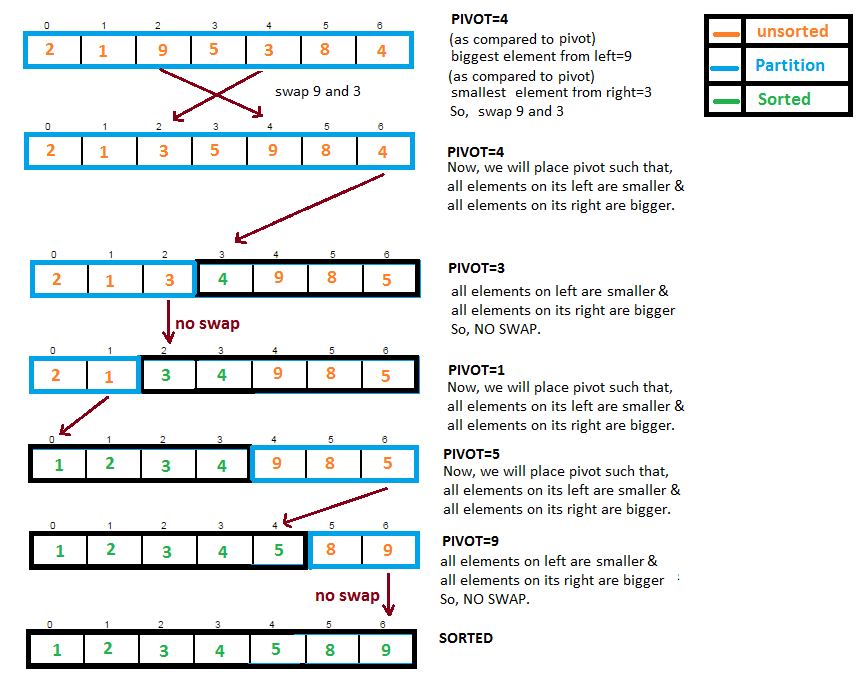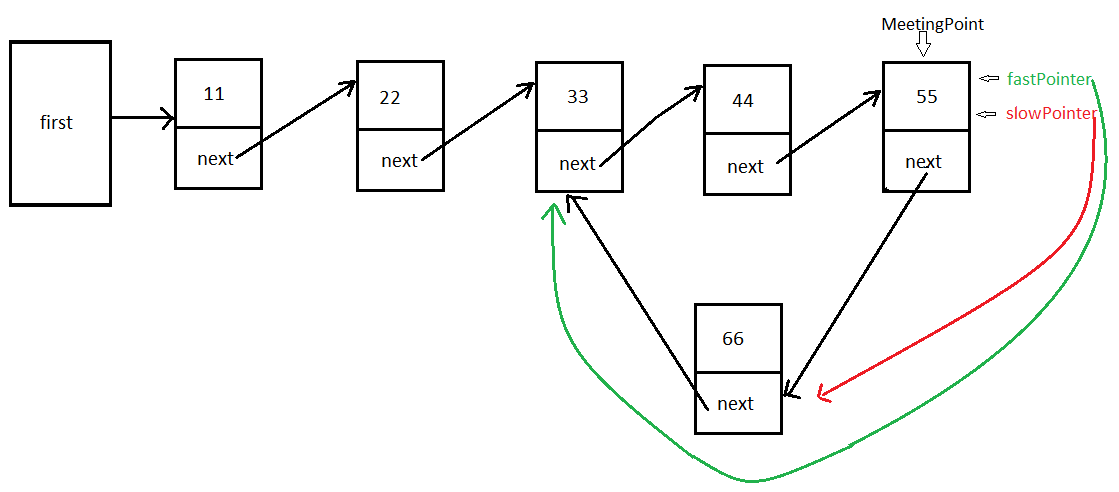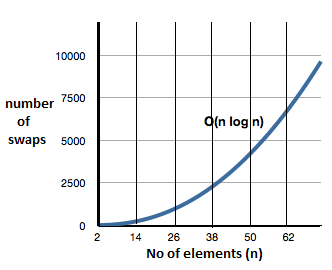### Quick sort with complexity explanation and diagram in java

Contents of page >
• How Quicksort works in java?
• Quicksort in diagram-
• Full Program/SourceCode/Example of QuickSort in java >
• Complexity of Quicksort in java >
• Comparisons required in Quicksort  in java>
• Complexity of Quicksort in graph >

How Quicksort works in java?

Quicksort is one the most popular sorting algorithm, even it is used in java API’s at many places. It offers time complexity of O(N*logN).

>Pick the Pivot (right most element) in array.
>place pivot such that,
all elements in its left are smaller &
all elements in its right are larger.
>After placing pivot, left & right partitions are formed.

Again pick the pivot, recursively we divide the partitions further into  left & right partitions  until array is completely sorted.

Quicksort in diagram-

Initially whole array is one partition, once pivot is placed at its position two partitions are formed, and we repeat the above procedure.Calculate nodes in circular Single LinkedList.

Full Program/SourceCode/Example of Quick Sort in java >
 /** Copyright (c), AnkitMittal www.JavaMadeSoEasy.com */ public class QuickSort {    static int[] ar = { 2, 1, 9, 5, 3, 8, 4 };    public static void main(String[] args) {                     System.out.print("Display array before Quick sorting: "  );           for (int i = 0; i < ar.length; i++)                  System.out.print(ar[i] +" ");                     quickSort(0, ar.length - 1);                     System.out.print("\nDisplay array after Quick sorting: "  );           for (int i = 0; i < ar.length; i++)                  System.out.print(ar[i] +" ");              }          /*    * method performs quick sort in java.    */    public static void quickSort(int left, int right) {           if (right - left <= 0) // size <= 1,                  return; // means array is already sorted.           else{                  int pivot = ar[right]; // rightmost item                  int partition = partitionArray(pivot, left, right); //find out partition range.                  quickSort(left, partition - 1);   // sort the left side.                  quickSort(partition + 1, right); // sort the right side.           }    }       //partition of array in java    public static  int partitionArray(int pivot, int left, int right) {           int leftIndicator = left - 1;           int rightIndicator = right;           while (true) {                  // find bigger element.                  while(ar[++leftIndicator] < pivot);          //execute while loop till -> elements are smaller than pivot, as soon as bigger element is found we stop while loop.                            // find smaller element.                  while(rightIndicator>0 && ar[--rightIndicator]>pivot);//execute while loop till -> elements are greater than pivot, as soon as smaller element is found we stop while loop.                                         if (leftIndicator >= rightIndicator) // if pointers have crossed, partition have been done, break.                        break;                  else                        swapElements(leftIndicator, rightIndicator); //If we haven't crossed swap the elements.           }                     swapElements(leftIndicator, right); // restoring the pivot.           return leftIndicator; //return restored pivot location.    }       public static void swapElements(int pos1, int pos2){           int temp = ar[pos1];           ar[pos1] = ar[pos2];           ar[pos2] = temp;    }    } /*OUTPUT Display array before Quick sorting: 2 1 9 5 3 8 4 Display array after Quick sorting: 1 2 3 4 5 8 9 */

So, we wrote program to do quick Sort of array in java.

Complexity of Quicksort in java >
Best,average and worst case >

Best Case :         O(N*logN) , though no of swaps may be 0 (if array is already sorted)
Average Case :  O(N*logN).
Worst Case :      O(N2),  (if array is reversely sorted)

Comparisons required in Quicksort  in java>

 N (number of elements) O(logN) O(N*logN) 8 3 24 16 4 64 32 5 160 64 6 384 128 7 896 256 8 2048

Complexity of Quicksort in graph >Summary >
So in this data structure tutorial we learned how Quicksort works in java. Quicksort in diagram. Program/Example of QuickSort in java. Complexity of Quicksort in java. Comparisons required in Quicksort  in java. Complexity of Quicksort in graph.

Having any doubt? or you you liked the tutorial! Please comment in below section.